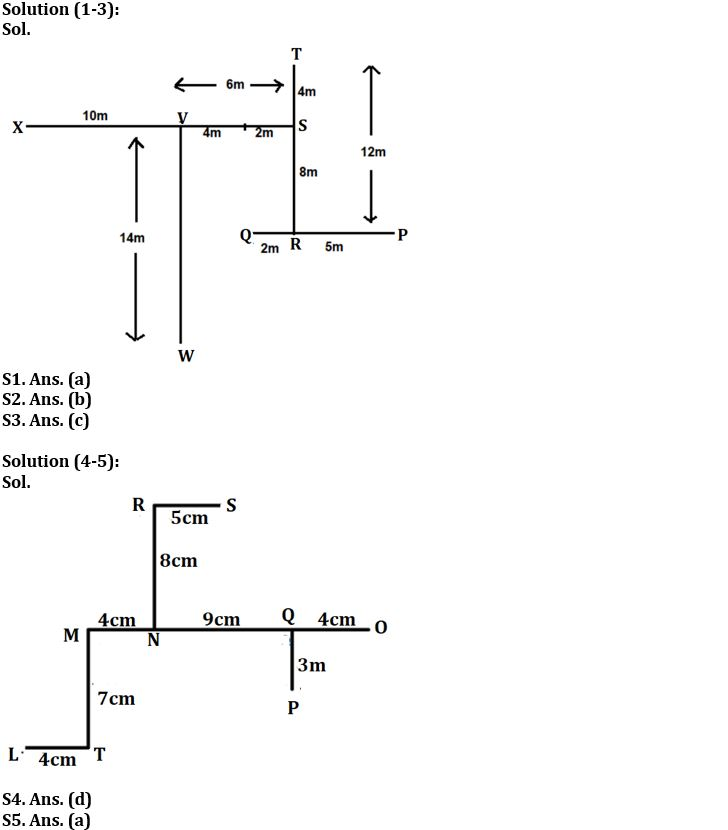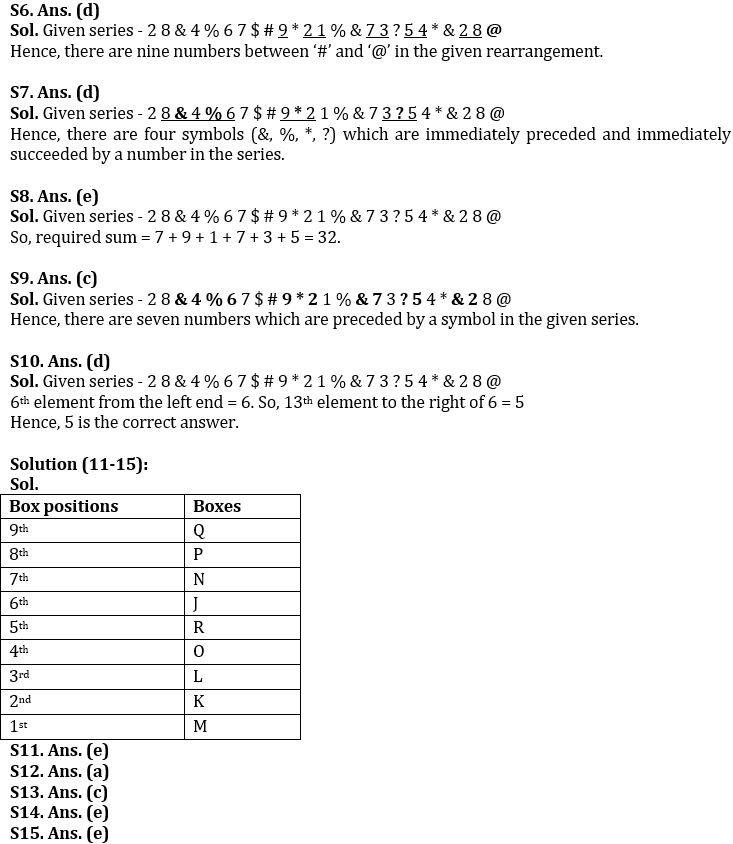Latest Banking jobs   »

# Reasoning Ability Quiz For IBPS Clerk/PO Prelims 2022- 23rd August

Directions (1-3): Answer the questions based on the information given below.
Point Q is 7m to the west of point P. Point R is 12m to the south of point T. Point P is 5m to the east of point R. Point S is 8m to the north of point R. Point V is 6m to the west of point S. Point W is 14m to the south of point V. Point V is 10m east of point X.

Q1. Point X is in which direction and how far with respect to the point S?
(a) 16m, west
(b) 16m, east
(c) 10m, east
(d) 12m, east
(e) 14m, west

Q2. Point W is in which direction with respect to Point T?
(a) South-east
(b) South-west
(c) North-east
(d) North-west
(e) None of the above

Q3. If Kunal sits 4m to the west of point Q, then what will be the shortest distance that Kunal has to cover to reach point S also in which direction?
(a) 10m, north
(b) 12m, south
(c) 10m, north-east
(d) 12m, south-west
(e) None of the above

Directions (4-5): Answer the questions based on the information given below.
Nine points are drawn on a rectangular paper. M is 7cm north of T. L is 4cm west of T. N is 4cm east of M. P is 3cm south of Q. O is 4cm east of Q. S is east of R. N is 8cm south of R. Q is 9cm east of N. The distance between R and S is equal to the shortest distance between O and P.

Q4. Point S is in which direction with respect to Point Q?
(a) South-east
(b) South-west
(c) North-east
(d) North-west
(e) None of the above

Q5. Point P is in which direction with respect to Point M?
(a) South-east
(b) South-west
(c) North-east
(d) North-west
(e) None of the above

Directions (6-10): Read the given series of numbers and symbols carefully and answer the questions based on it.

2 8 & 4 % 6 7 \$ # 9 * 2 1 % & 7 3 ? 5 4 * & 2 8 @

Q6. How many numbers are between ‘#’ and ‘@’ in the given series?
(a) Seven
(b) Six
(c) Eight
(d) Nine
(e) None of the above

Q7. How many symbols are immediately preceded and immediately succeeded by a number in the series?
(a) Two
(b) Six
(c) Three
(d) Four
(e) None of the above

Q8. What will be the sum of the all-odd numbers in the given series?
(a) 28
(b) 39
(c) 40
(d) 30
(e) 32

Q9. How many numbers are preceded by a symbol in the given series?
(a) Eight
(b) Six
(c) Seven
(d) Four
(e) None of the above

Q10. Which is 13th element to the right of 6th element from the left end in the given series?
(a) *
(b) 4
(c) &
(d) 5
(e) None of the above

Directions (11-15): Answer the questions based on the information given below.

Nine boxes (J, K, L, M, N, O, P, Q, and R) are kept one above the other, but not necessarily in the same order. The bottommost box is numbered as 1 and the topmost box is numbered as 9.
N is kept 3rd to the above of O. O is 4th box. P which is a perfect cube numbered box (but not 1), is kept immediately below Q. Two boxes are kept between L and J. J is kept adjacent to N. M is kept immediately below K. K is not an odd numbered box.

Q11. Which of the following is 2nd box?
(a) M
(b) Q
(c) R
(d) L
(e) None of the above

Q12. R is kept ___________ to the below of Q.
(a) 4th
(b) 2nd
(c) 3rd
(d) 5th
(e) None of the above

Q13. Which box is kept immediately above L?
(a) K
(b) M
(c) O
(d) R
(e) None of the above

Q14. How many boxes are kept below R?
(a) Five
(b) Two
(c) One
(d) None
(e) None of the above

Q15. Which of the following is not a prime numbered box?
(a) N
(b) R
(c) K
(d) L
(e) J

Solutions#### Congratulations!Download Hindu Review of October 2021: Free PDF